# Distillation Underlying Principles of Distillation Azeotropes and VLE

• Slides: 17
Download presentationDistillation Underlying Principles of Distillation Azeotropes and VLE Data Gavin Duffy School of Electrical Engineering Systems DIT Kevin Street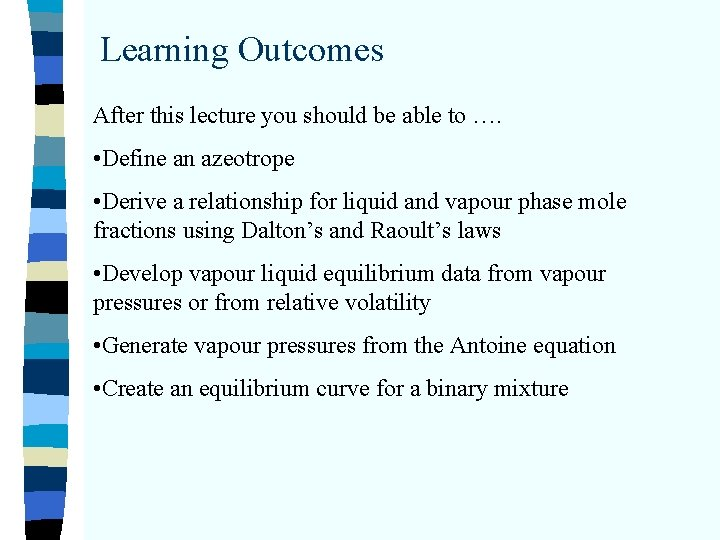Learning Outcomes After this lecture you should be able to …. • Define an azeotrope • Derive a relationship for liquid and vapour phase mole fractions using Dalton’s and Raoult’s laws • Develop vapour liquid equilibrium data from vapour pressures or from relative volatility • Generate vapour pressures from the Antoine equation • Create an equilibrium curve for a binary mixture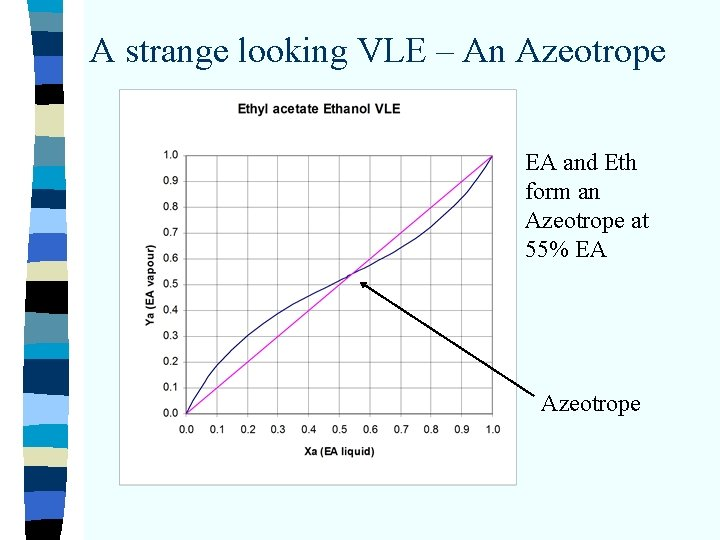A strange looking VLE – An Azeotrope EA and Eth form an Azeotrope at 55% EA Azeotrope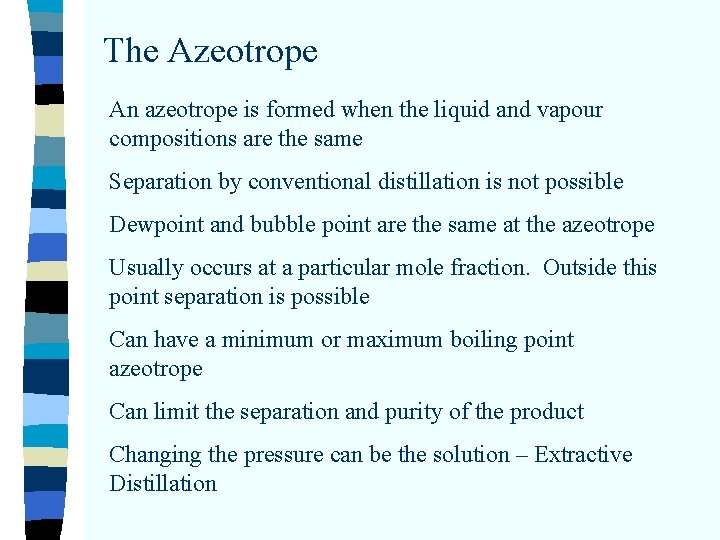The Azeotrope An azeotrope is formed when the liquid and vapour compositions are the same Separation by conventional distillation is not possible Dewpoint and bubble point are the same at the azeotrope Usually occurs at a particular mole fraction. Outside this point separation is possible Can have a minimum or maximum boiling point azeotrope Can limit the separation and purity of the product Changing the pressure can be the solution – Extractive DistillationMin BP Azeotrope – EA Ethanol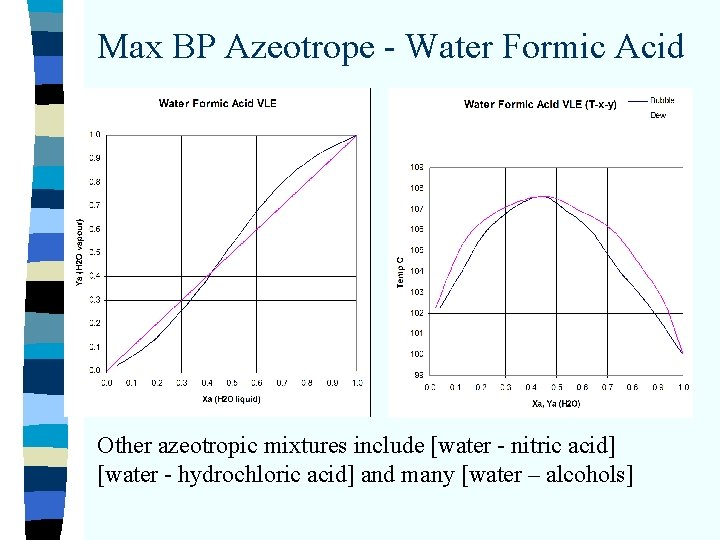Max BP Azeotrope - Water Formic Acid Other azeotropic mixtures include [water - nitric acid] [water - hydrochloric acid] and many [water – alcohols]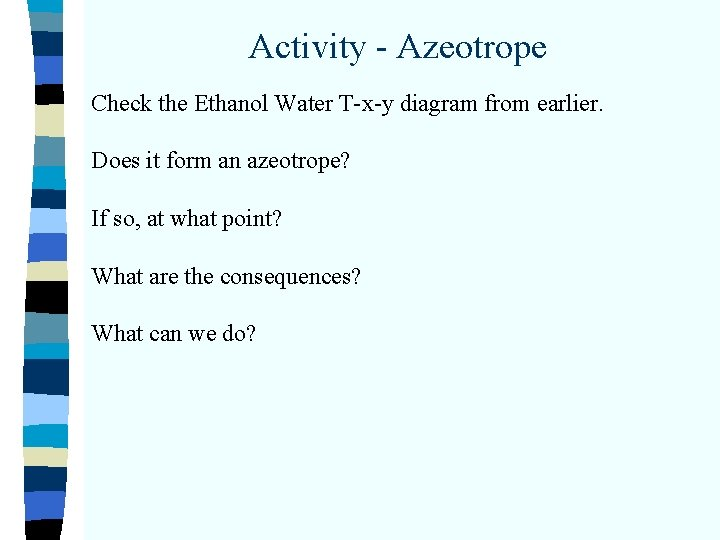Activity - Azeotrope Check the Ethanol Water T-x-y diagram from earlier. Does it form an azeotrope? If so, at what point? What are the consequences? What can we do?When equilibrium data are not available How can you decide if distillation will be a suitable separation technique if you don’t have T-x-y data? You have to make your own! Oh No! Can be difficult We need vapour pressures. These are obtained by • Looking them up in a book • Calculating them • Use VP’s from book and construct a graph of VP v T • From VP’s we can determine mole fractions The vapour pressures are needed at specific temperatures so they can be hard to find in the books.1. Partial Pressure, Dalton and Raoult Dalton’s Law Pa = ya. P Pa is the partial pressure, ya is the vapour mole fraction and P is the total pressure Raoult’s law – applies to an ideal mixture Pa = Poaxa Poa is the vapour pressure, xa is the liquid mole fraction We assume we are dealing with ideal mixtures.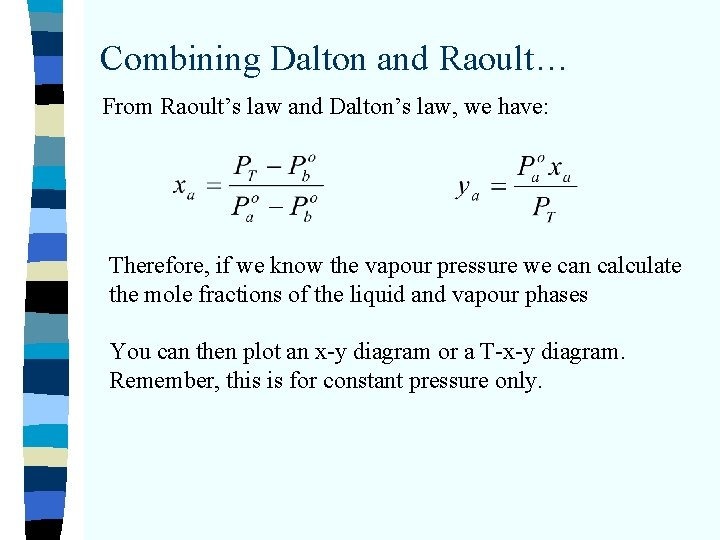Combining Dalton and Raoult… From Raoult’s law and Dalton’s law, we have: Therefore, if we know the vapour pressure we can calculate the mole fractions of the liquid and vapour phases You can then plot an x-y diagram or a T-x-y diagram. Remember, this is for constant pressure only.2. From Relative Volatility This is another way to get mole fractions. We can determine the relative volatility from the Vapour Pressures. Use the definition of relative volatility and Raoult’s law to get the following: is a function of VP which is a function of T. (If you need just one then take the average of at the two b. p. ’s). Next, include Dalton’s law to get an expression for mole fractions: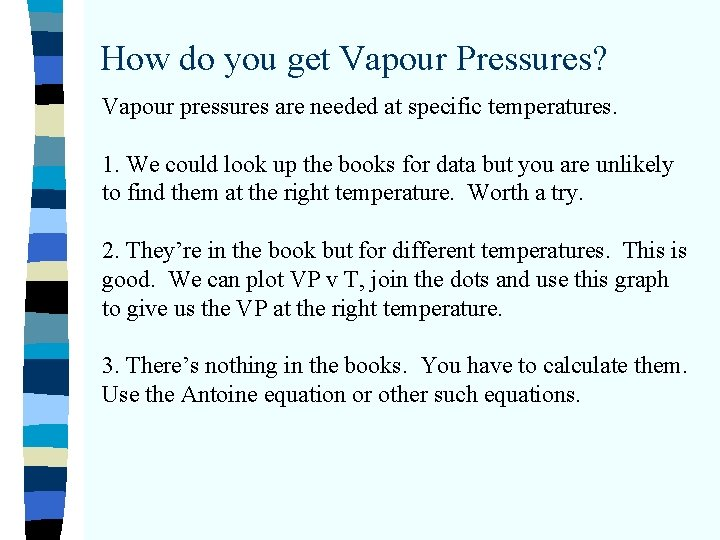How do you get Vapour Pressures? Vapour pressures are needed at specific temperatures. 1. We could look up the books for data but you are unlikely to find them at the right temperature. Worth a try. 2. They’re in the book but for different temperatures. This is good. We can plot VP v T, join the dots and use this graph to give us the VP at the right temperature. 3. There’s nothing in the books. You have to calculate them. Use the Antoine equation or other such equations.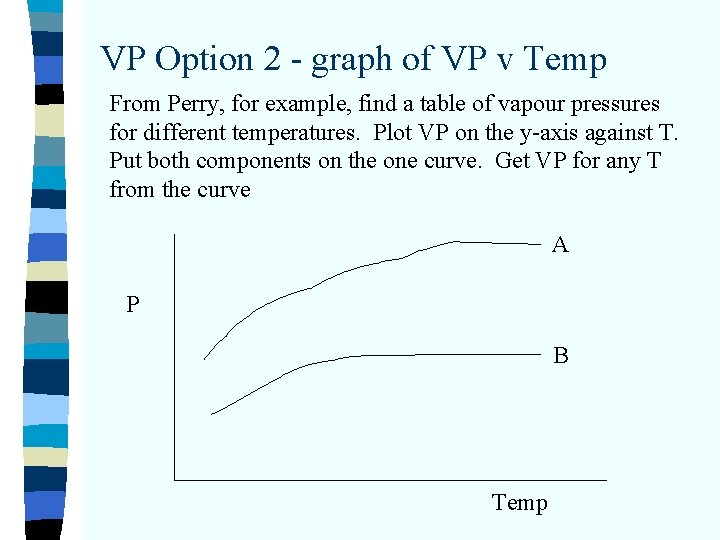VP Option 2 - graph of VP v Temp From Perry, for example, find a table of vapour pressures for different temperatures. Plot VP on the y-axis against T. Put both components on the one curve. Get VP for any T from the curve A P B Temp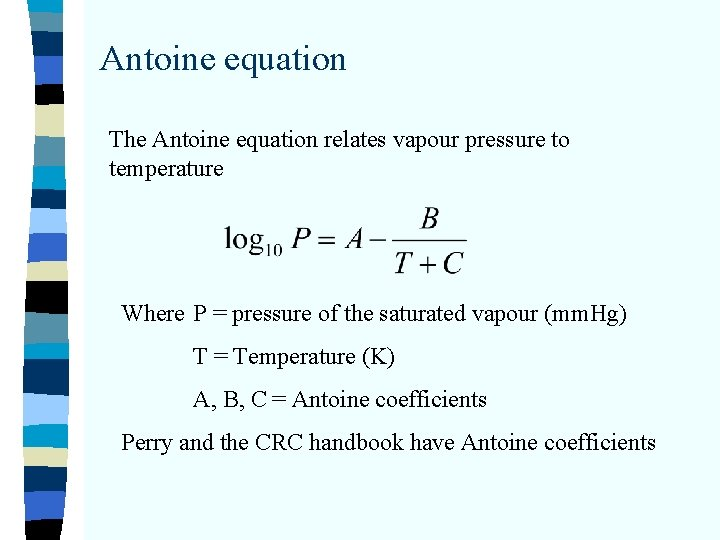Antoine equation The Antoine equation relates vapour pressure to temperature Where P = pressure of the saturated vapour (mm. Hg) T = Temperature (K) A, B, C = Antoine coefficients Perry and the CRC handbook have Antoine coefficients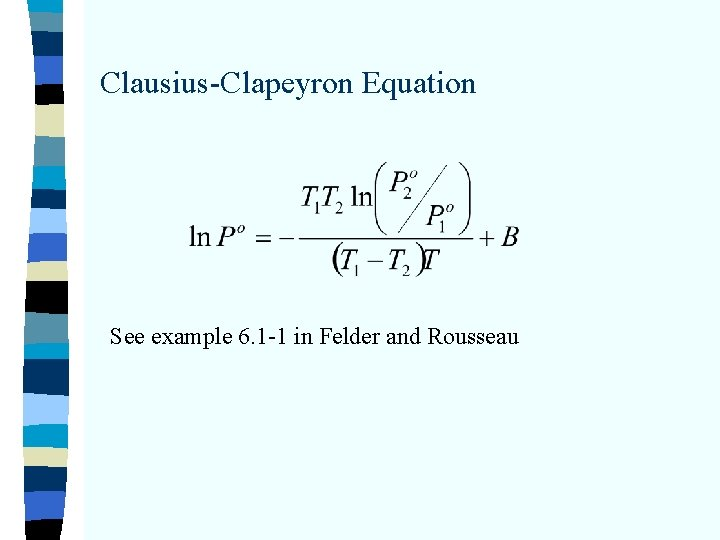Clausius-Clapeyron Equation See example 6. 1 -1 in Felder and Rousseau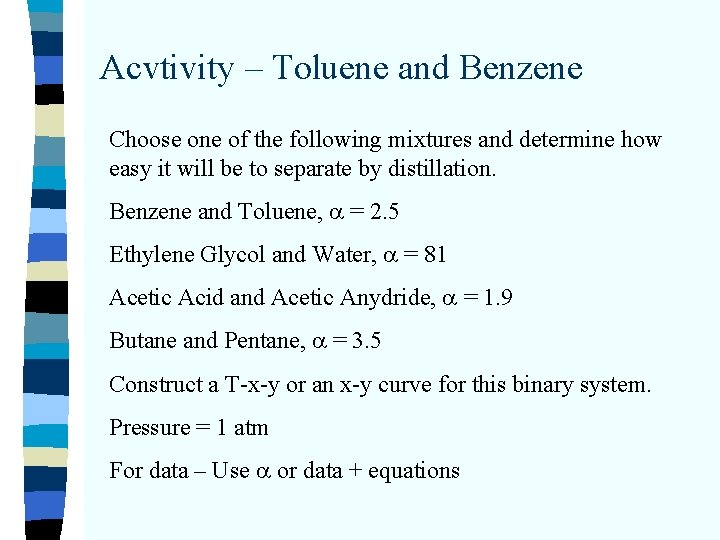Acvtivity – Toluene and Benzene Choose one of the following mixtures and determine how easy it will be to separate by distillation. Benzene and Toluene, = 2. 5 Ethylene Glycol and Water, = 81 Acetic Acid and Acetic Anydride, = 1. 9 Butane and Pentane, = 3. 5 Construct a T-x-y or an x-y curve for this binary system. Pressure = 1 atm For data – Use or data + equations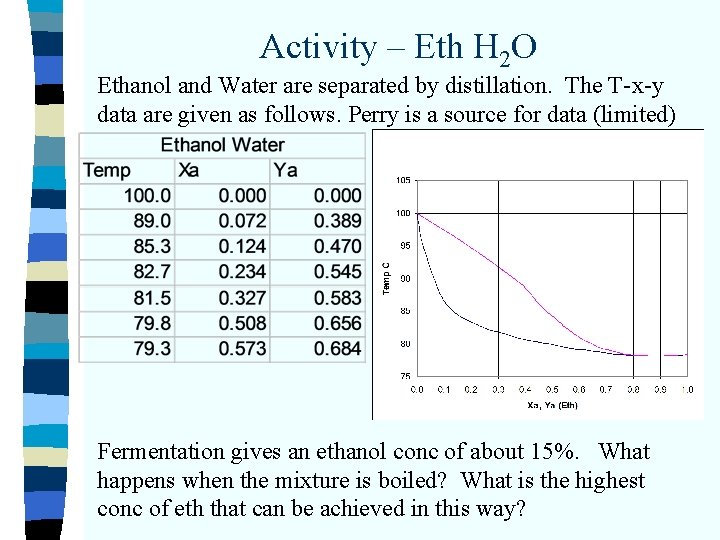Activity – Eth H 2 O Ethanol and Water are separated by distillation. The T-x-y data are given as follows. Perry is a source for data (limited) Fermentation gives an ethanol conc of about 15%. What happens when the mixture is boiled? What is the highest conc of eth that can be achieved in this way?